# Frank Solutions for Class 10 Maths Chapter 18 Constructions

Frank Solutions for Class 10 Maths Chapter 18 Constructions are available here so that students can refer to the solutions whenever they are facing difficulty while solving the questions. These solutions for Chapter 18 are available in PDF format, and students can download them and learn offline as well. The solutions are one of the top materials when it comes to providing a question bank for practice.

We, at BYJU’S, have prepared these Solutions for Class 10 Maths Chapter 18. The problems are formulated by our subject experts to assist you with your exam preparation to attain good marks in Maths. Download the PDF of Frank Solutions for Class 10 Maths Chapter 18 from the link given below.

## Download the PDF of Frank Solutions for Class 10 Maths Chapter 18 Constructions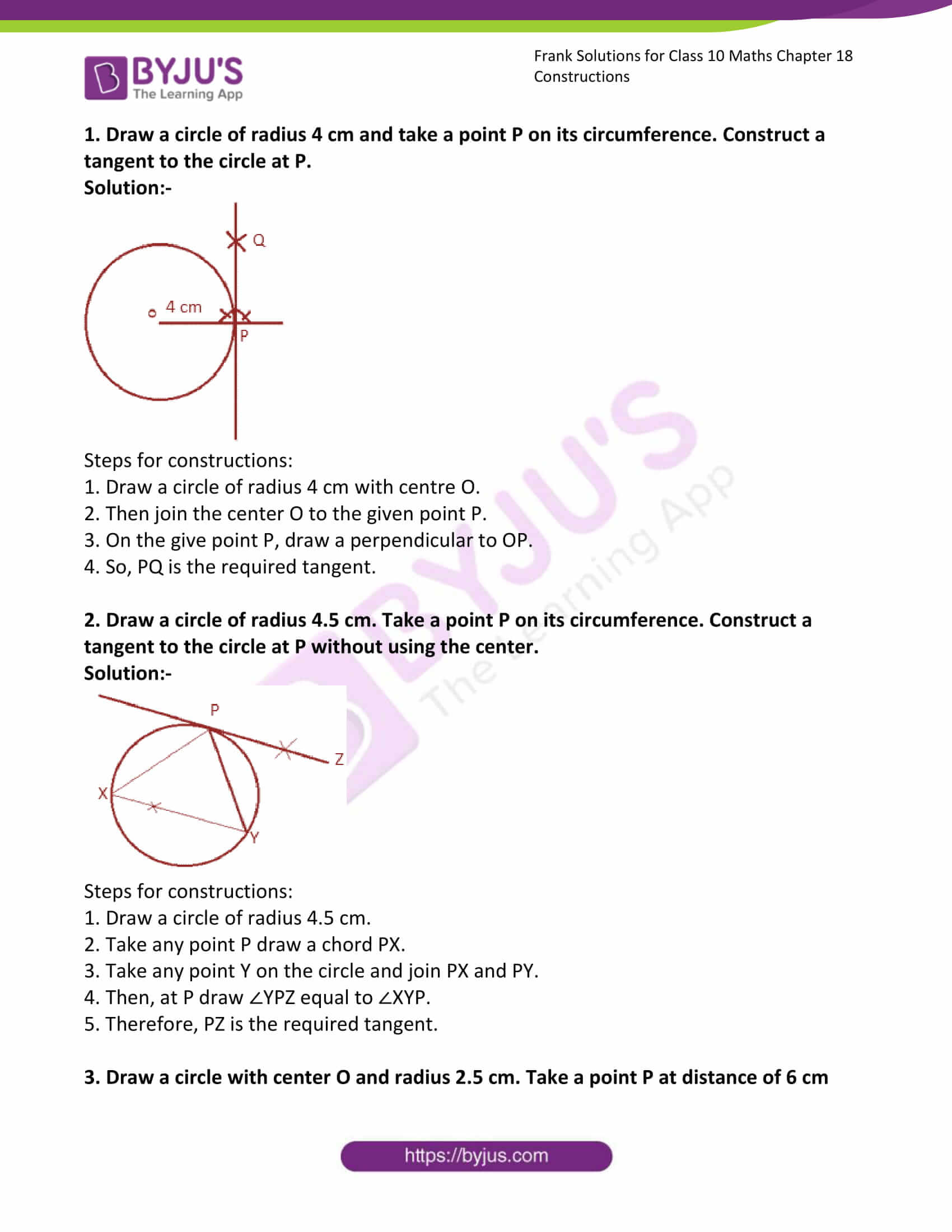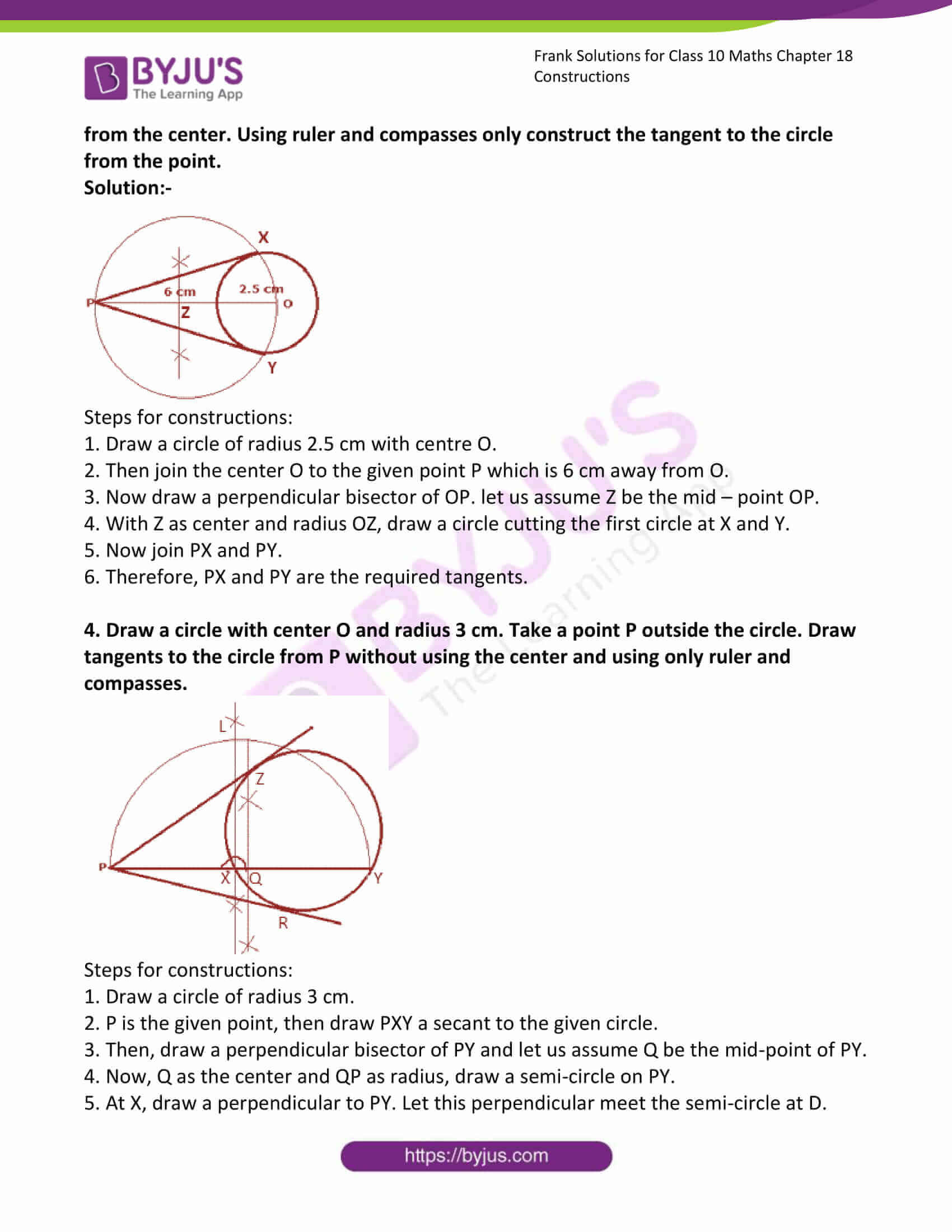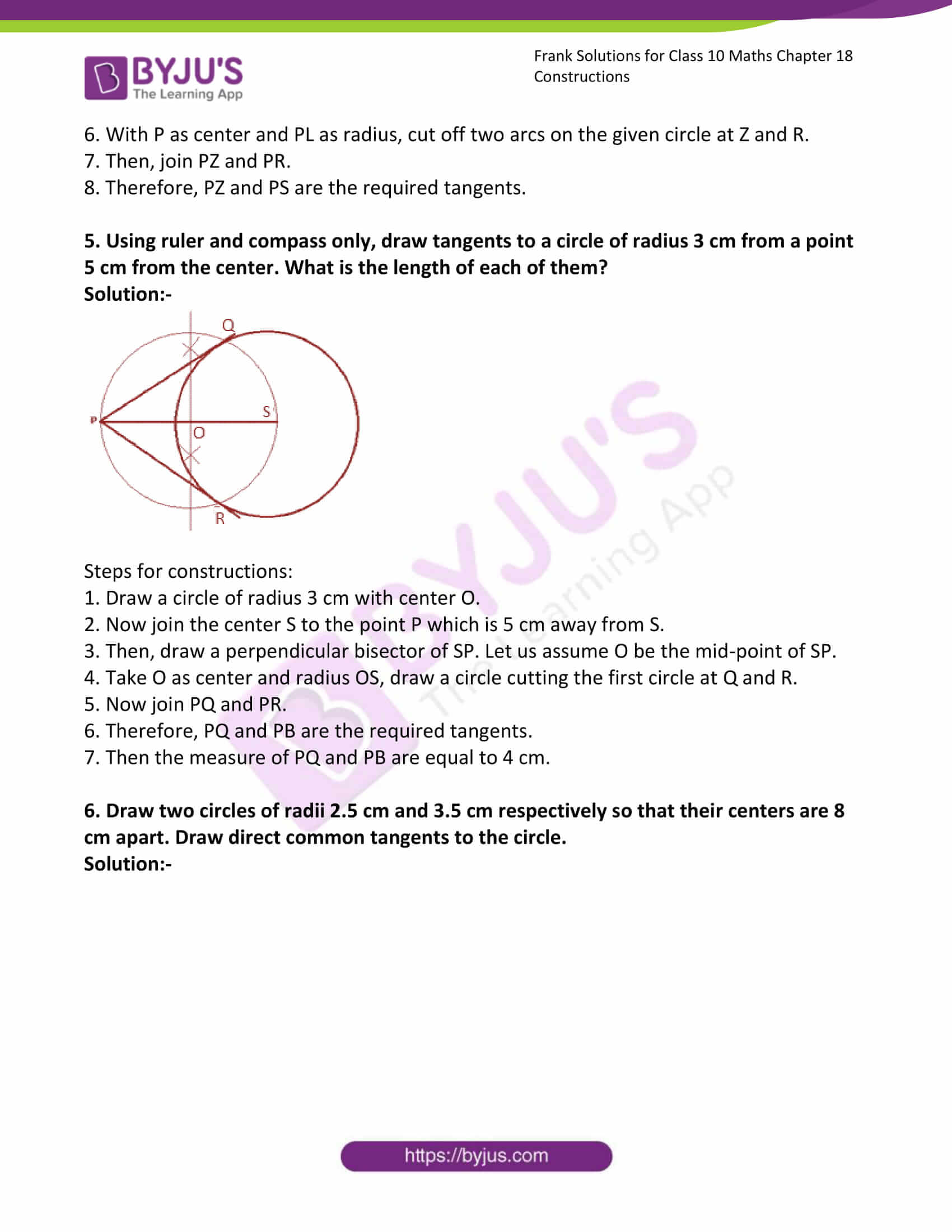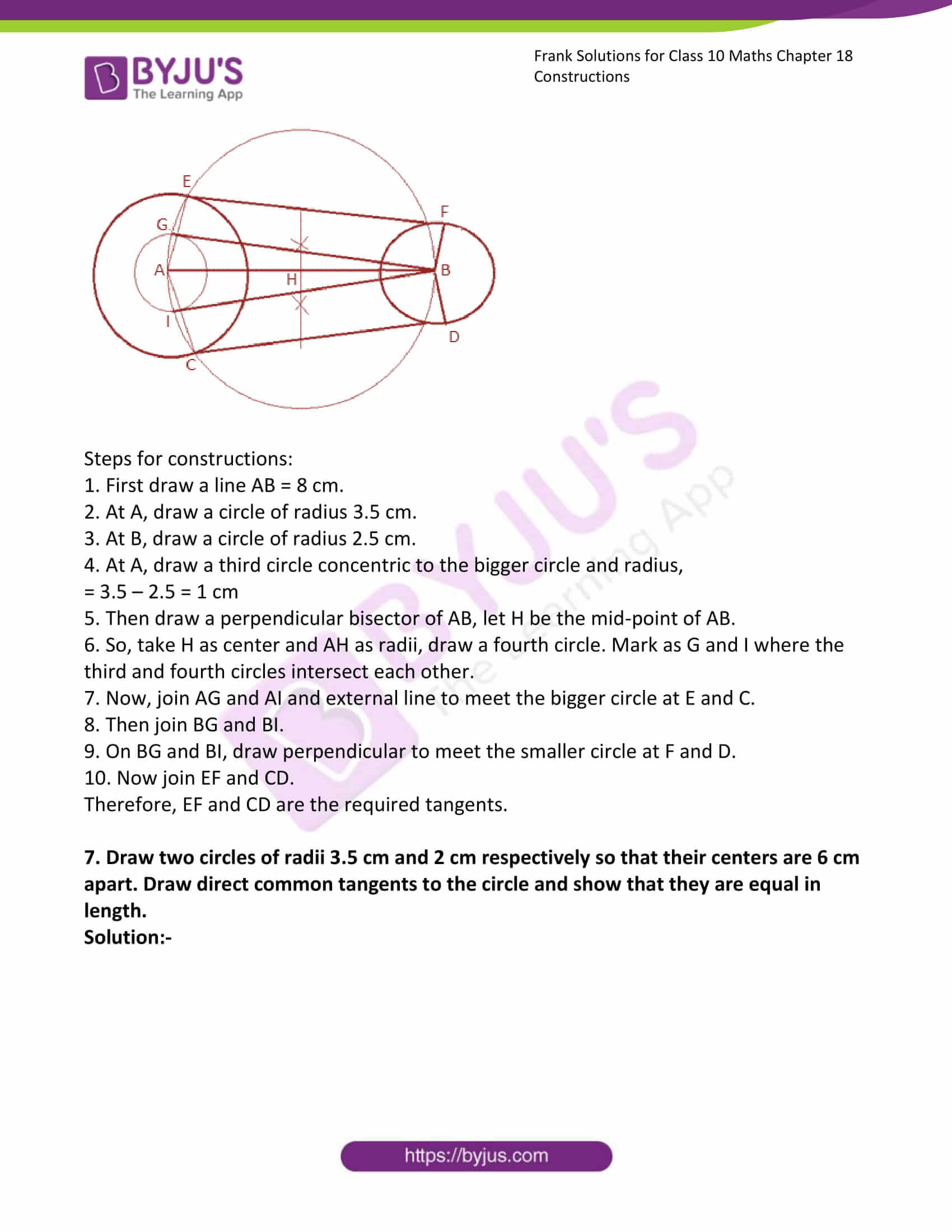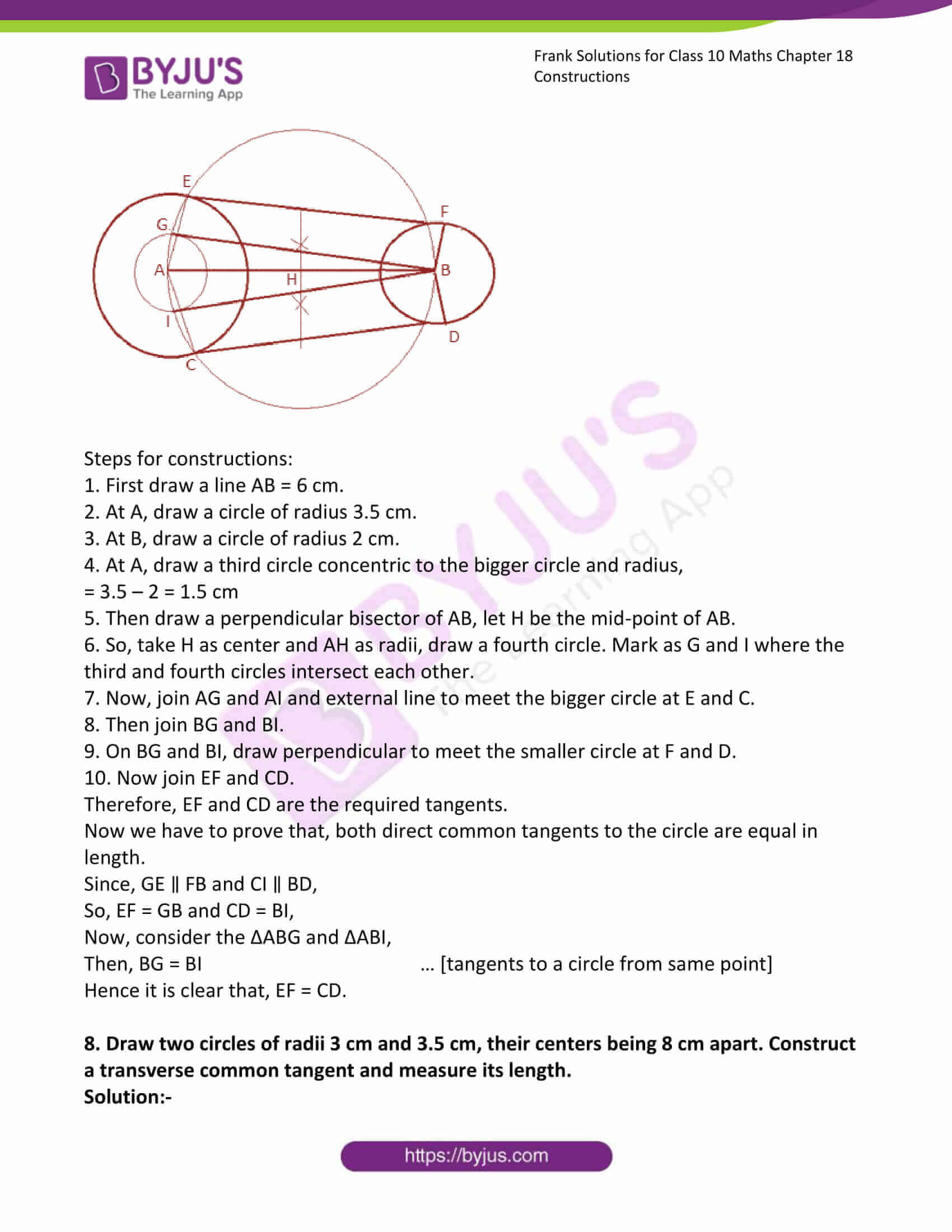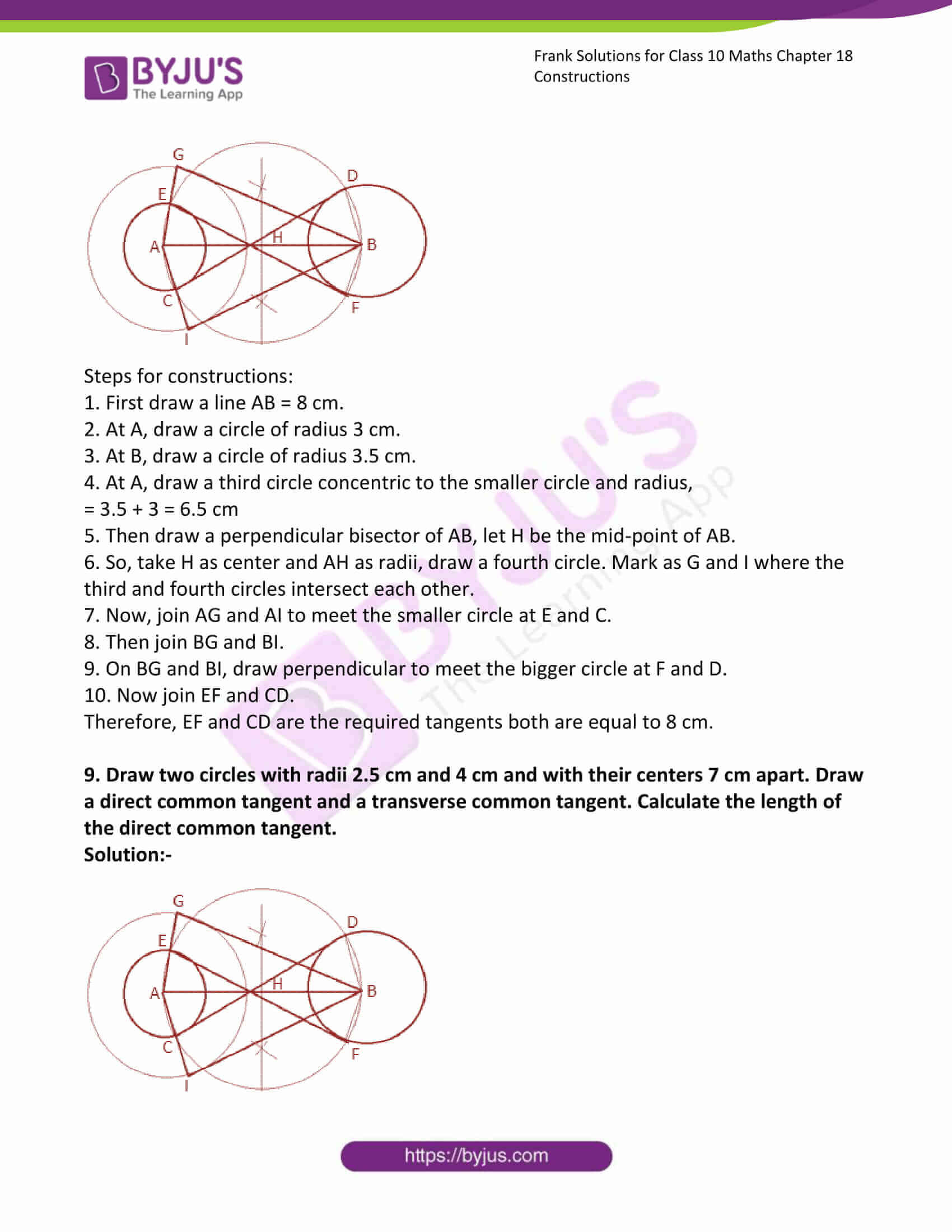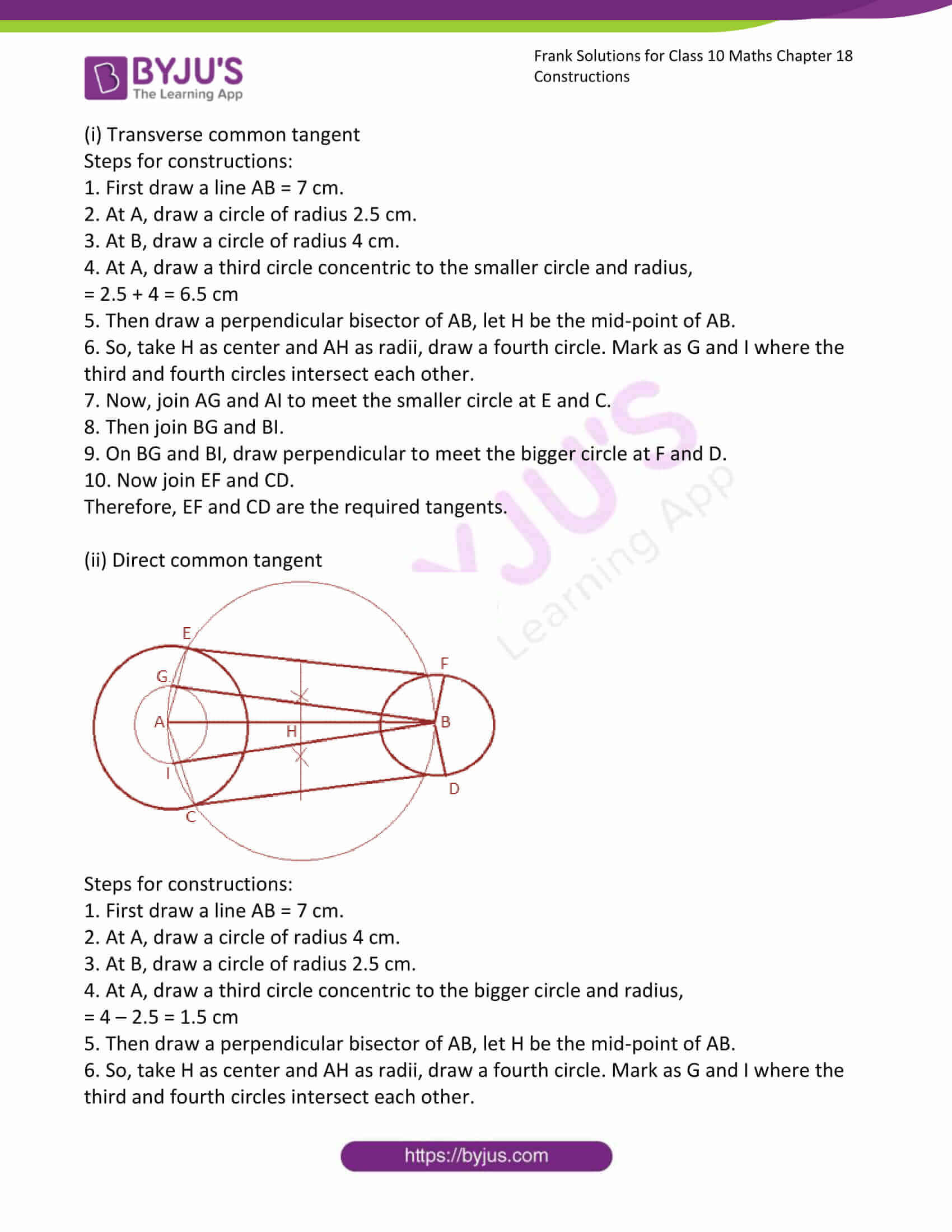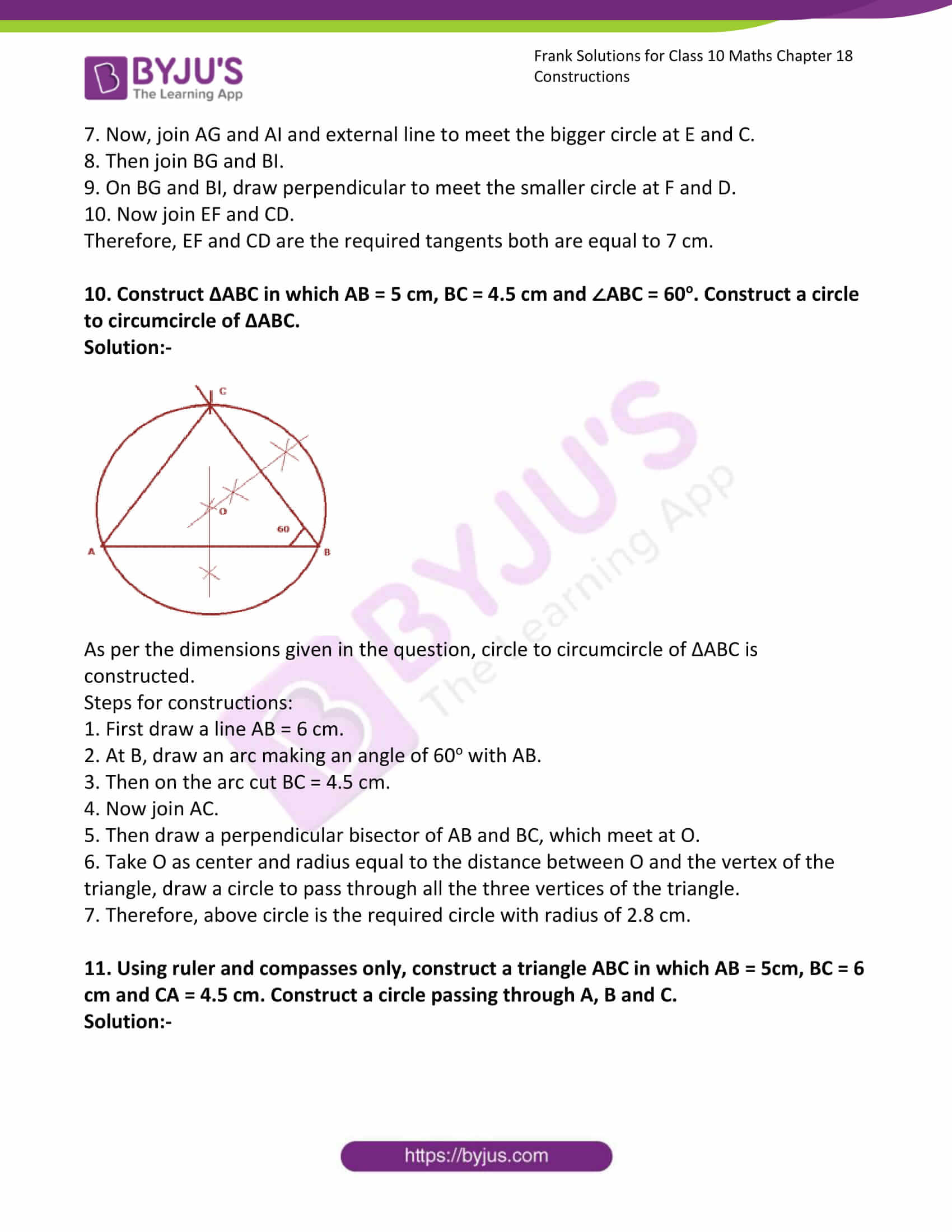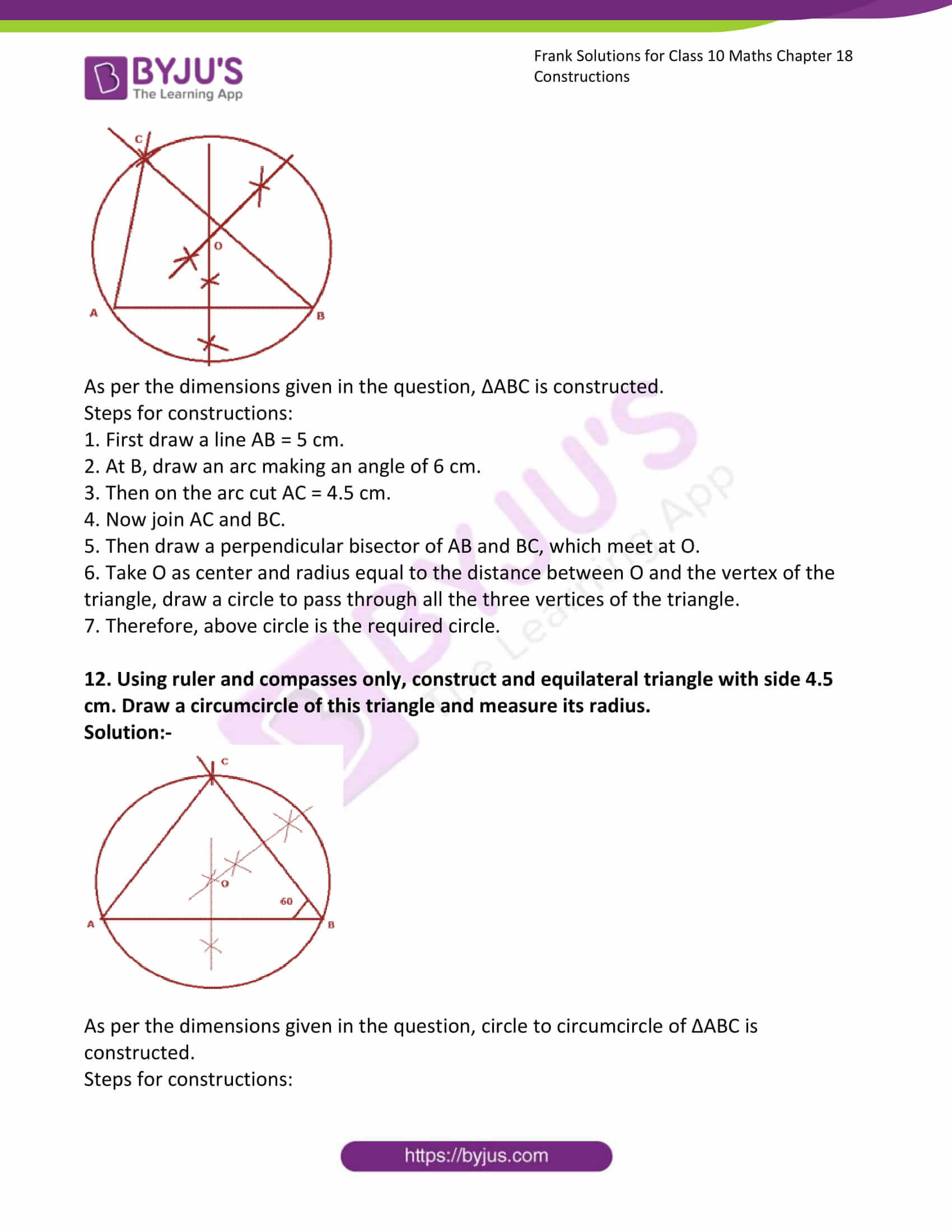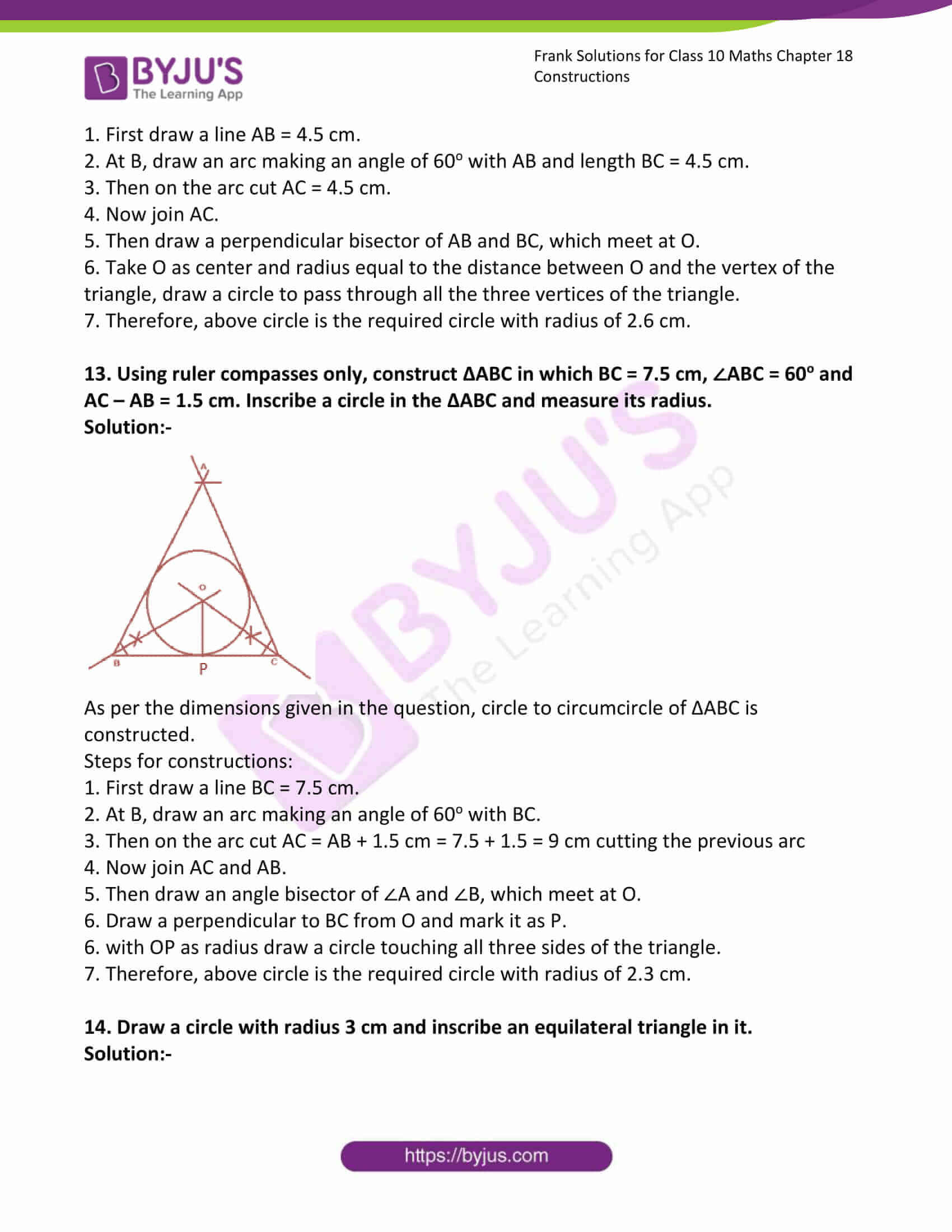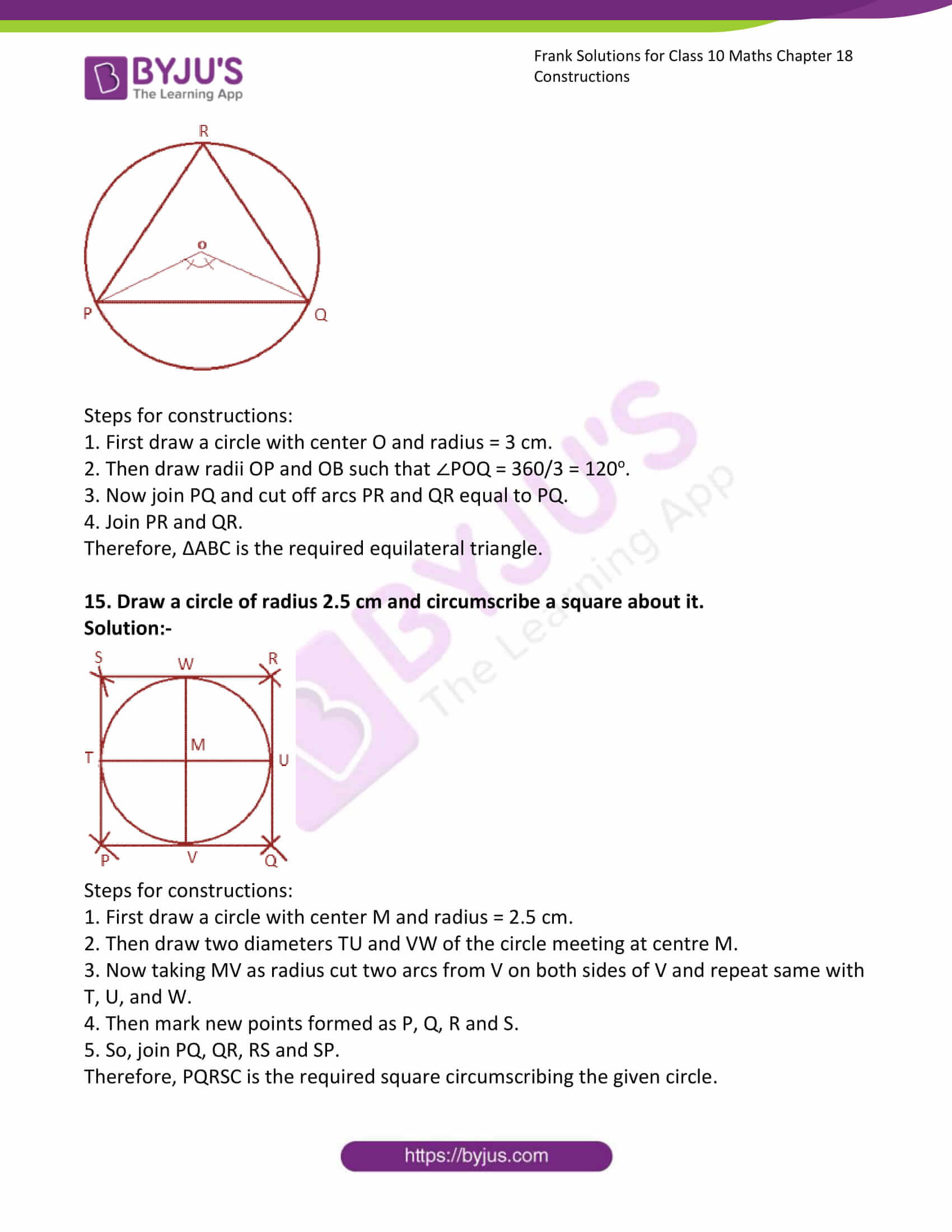### Access Answers to Frank Solutions for Class 10 Maths Chapter 18 Constructions

1. Draw a circle of radius 4 cm and take a point P on its circumference. Construct a tangent to the circle at P.

Solution:-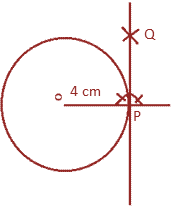Steps for constructions:

1. Draw a circle of radius 4 cm with centre O.

2. Then join the centre O to the given point P.

3. On the give point P, draw a perpendicular to OP.

4. So, PQ is the required tangent.

2. Draw a circle of radius 4.5 cm. Take a point P on its circumference. Construct a tangent to the circle at P without using the centre.

Solution:-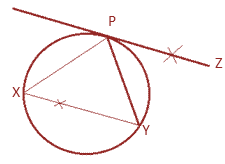Steps for constructions:

1. Draw a circle of radius 4.5 cm.

2. Take any point P draw a chord PX.

3. Take any point Y on the circle and join PX and PY.

4. Then, at P draw ∠YPZ equal to ∠XYP.

5. Therefore, PZ is the required tangent.

3. Draw a circle with centre O and radius 2.5 cm. Take a point P at distance of 6 cm from the centre. Using ruler and compasses only construct the tangent to the circle from the point.

Solution:-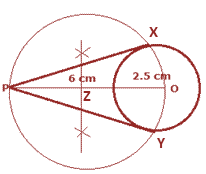Steps for constructions:

1. Draw a circle of radius 2.5 cm with centre O.

2. Then join the centre O to the given point P, which is 6 cm away from O.

3. Now draw a perpendicular bisector of OP. let us assume Z be the mid – point OP.

4. With Z as centre and radius OZ, draw a circle cutting the first circle at X and Y.

5. Now join PX and PY.

6. Therefore, PX and PY are the required tangents.

4. Draw a circle with centre O and radius 3 cm. Take a point P outside the circle. Draw tangents to the circle from P without using the centre and using only ruler and compasses.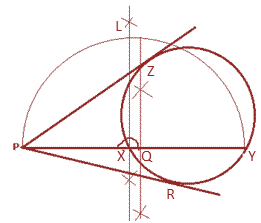Steps for constructions:

1. Draw a circle of radius 3 cm.

2. P is the given point, then draw PXY a secant to the given circle.

3. Then, draw a perpendicular bisector of PY and let us assume Q be the mid-point of PY.

4. Now, Q as the centre and QP as radius, draw a semi-circle on PY.

5. At X, draw a perpendicular to PY. Let this perpendicular meet the semi-circle at D.

6. With P as centre and PL as radius, cut off two arcs on the given circle at Z and R.

7. Then, join PZ and PR.

8. Therefore, PZ and PS are the required tangents.

5. Using ruler and compass only, draw tangents to a circle of radius 3 cm from a point 5 cm from the centre. What is the length of each of them?

Solution:-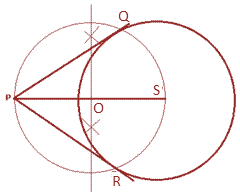Steps for constructions:

1. Draw a circle of radius 3 cm with centre O.

2. Now join the centre S to the point P which is 5 cm away from S.

3. Then, draw a perpendicular bisector of SP. Let us assume O be the mid-point of SP.

4. Take O as centre and radius OS, draw a circle cutting the first circle at Q and R.

5. Now join PQ and PR.

6. Therefore, PQ and PB are the required tangents.

7. Then the measure of PQ and PB are equal to 4 cm.

6. Draw two circles of radii 2.5 cm and 3.5 cm respectively so that their centres are 8 cm apart. Draw direct common tangents to the circle.

Solution:-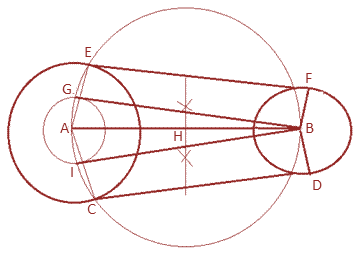Steps for constructions:

1. First draw a line AB = 8 cm.

2. At A, draw a circle of radius 3.5 cm.

3. At B, draw a circle of radius 2.5 cm.

4. At A, draw a third circle concentric to the bigger circle and radius,

= 3.5 – 2.5 = 1 cm

5. Then draw a perpendicular bisector of AB, let H be the mid-point of AB.

6. So, take H as centre and AH as radii, draw a fourth circle. Mark as G and I where the third and fourth circles intersect each other.

7. Now, join AG and AI and external line to meet the bigger circle at E and C.

8. Then join BG and BI.

9. On BG and BI, draw perpendicular to meet the smaller circle at F and D.

10. Now join EF and CD.

Therefore, EF and CD are the required tangents.

7. Draw two circles of radii 3.5 cm and 2 cm respectively so that their centres are 6 cm apart. Draw direct common tangents to the circle and show that they are equal in length.

Solution:-Steps for constructions:

1. First draw a line AB = 6 cm.

2. At A, draw a circle of radius 3.5 cm.

3. At B, draw a circle of radius 2 cm.

4. At A, draw a third circle concentric to the bigger circle and radius,

= 3.5 – 2 = 1.5 cm

5. Then draw a perpendicular bisector of AB, let H be the mid-point of AB.

6. So, take H as centre and AH as radii, draw a fourth circle. Mark as G and I where the third and fourth circles intersect each other.

7. Now, join AG and AI and external line to meet the bigger circle at E and C.

8. Then join BG and BI.

9. On BG and BI, draw perpendicular to meet the smaller circle at F and D.

10. Now join EF and CD.

Therefore, EF and CD are the required tangents.

Now we have to prove that, both direct common tangents to the circle are equal in length.

Since, GE ∥ FB and CI ∥ BD,

So, EF = GB and CD = BI,

Now, consider the ΔABG and ΔABI,

Then, BG = BI … [tangents to a circle from same point]

Hence it is clear that, EF = CD.

8. Draw two circles of radii 3 cm and 3.5 cm, their centres being 8 cm apart. Construct a transverse common tangent and measure its length.

Solution:-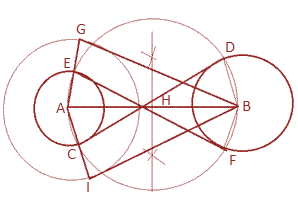Steps for constructions:

1. First draw a line AB = 8 cm.

2. At A, draw a circle of radius 3 cm.

3. At B, draw a circle of radius 3.5 cm.

4. At A, draw a third circle concentric to the smaller circle and radius,

= 3.5 + 3 = 6.5 cm

5. Then draw a perpendicular bisector of AB, let H be the mid-point of AB.

6. So, take H as centre and AH as radii, draw a fourth circle. Mark as G and I where the third and fourth circles intersect each other.

7. Now, join AG and AI to meet the smaller circle at E and C.

8. Then join BG and BI.

9. On BG and BI, draw perpendicular to meet the bigger circle at F and D.

10. Now join EF and CD.

Therefore, EF and CD are the required tangents both are equal to 8 cm.

9. Draw two circles with radii 2.5 cm and 4 cm and with their centres 7 cm apart. Draw a direct common tangent and a transverse common tangent. Calculate the length of the direct common tangent.

Solution:-(i) Transverse common tangent

Steps for constructions:

1. First draw a line AB = 7 cm.

2. At A, draw a circle of radius 2.5 cm.

3. At B, draw a circle of radius 4 cm.

4. At A, draw a third circle concentric to the smaller circle and radius,

= 2.5 + 4 = 6.5 cm

5. Then draw a perpendicular bisector of AB, let H be the mid-point of AB.

6. So, take H as centre and AH as radii, draw a fourth circle. Mark as G and I where the third and fourth circles intersect each other.

7. Now, join AG and AI to meet the smaller circle at E and C.

8. Then join BG and BI.

9. On BG and BI, draw perpendicular to meet the bigger circle at F and D.

10. Now join EF and CD.

Therefore, EF and CD are the required tangents.

(ii) Direct common tangentSteps for constructions:

1. First draw a line AB = 7 cm.

2. At A, draw a circle of radius 4 cm.

3. At B, draw a circle of radius 2.5 cm.

4. At A, draw a third circle concentric to the bigger circle and radius,

= 4 – 2.5 = 1.5 cm

5. Then draw a perpendicular bisector of AB, let H be the mid-point of AB.

6. So, take H as centre and AH as radii, draw a fourth circle. Mark as G and I where the third and fourth circles intersect each other.

7. Now, join AG and AI and external line to meet the bigger circle at E and C.

8. Then join BG and BI.

9. On BG and BI, draw perpendicular to meet the smaller circle at F and D.

10. Now join EF and CD.

Therefore, EF and CD are the required tangents both are equal to 7 cm.

10. Construct ΔABC in which AB = 5 cm, BC = 4.5 cm and ∠ABC = 60o. Construct a circle to circumcircle of ΔABC.

Solution:-As per the dimensions given in the question, circle to circumcircle of ΔABC is constructed.

Steps for constructions:

1. First draw a line AB = 6 cm.

2. At B, draw an arc making an angle of 60o with AB.

3. Then on the arc cut BC = 4.5 cm.

4. Now join AC.

5. Then draw a perpendicular bisector of AB and BC, which meet at O.

6. Take O as centre and radius equal to the distance between O and the vertex of the triangle, draw a circle to pass through all the three vertices of the triangle.

7. Therefore, above circle is the required circle with radius of 2.8 cm.

11. Using ruler and compasses only, construct a triangle ABC in which AB = 5cm, BC = 6 cm and CA = 4.5 cm. Construct a circle passing through A, B and C.

Solution:-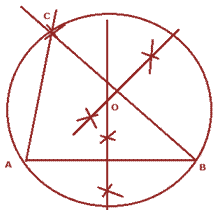As per the dimensions given in the question, ΔABC is constructed.

Steps for constructions:

1. First draw a line AB = 5 cm.

2. At B, draw an arc making an angle of 6 cm.

3. Then on the arc cut AC = 4.5 cm.

4. Now join AC and BC.

5. Then draw a perpendicular bisector of AB and BC, which meet at O.

6. Take O as centre and radius equal to the distance between O and the vertex of the triangle, draw a circle to pass through all the three vertices of the triangle.

7. Therefore, above circle is the required circle.

12. Using ruler and compasses only, construct and equilateral triangle with side 4.5 cm. Draw a circumcircle of this triangle and measure its radius.

Solution:-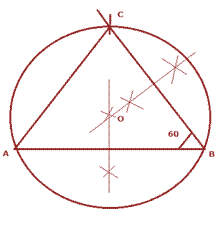As per the dimensions given in the question, circle to circumcircle of ΔABC is constructed.

Steps for constructions:

1. First draw a line AB = 4.5 cm.

2. At B, draw an arc making an angle of 60o with AB and length BC = 4.5 cm.

3. Then on the arc cut AC = 4.5 cm.

4. Now join AC.

5. Then draw a perpendicular bisector of AB and BC, which meet at O.

6. Take O as centre and radius equal to the distance between O and the vertex of the triangle, draw a circle to pass through all the three vertices of the triangle.

7. Therefore, above circle is the required circle with radius of 2.6 cm.

13. Using ruler compasses only, construct ΔABC in which BC = 7.5 cm, ∠ABC = 60o and AC – AB = 1.5 cm. Inscribe a circle in the ΔABC and measure its radius.

Solution:-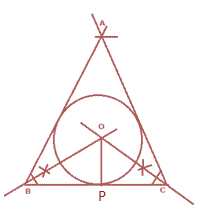As per the dimensions given in the question, circle to circumcircle of ΔABC is constructed.

Steps for constructions:

1. First draw a line BC = 7.5 cm.

2. At B, draw an arc making an angle of 60o with BC.

3. Then on the arc cut AC = AB + 1.5 cm = 7.5 + 1.5 = 9 cm cutting the previous arc

4. Now join AC and AB.

5. Then draw an angle bisector of ∠A and ∠B, which meet at O.

6. Draw a perpendicular to BC from O and mark it as P.

6. with OP as radius draw a circle touching all three sides of the triangle.

7. Therefore, above circle is the required circle with radius of 2.3 cm.

14. Draw a circle with radius 3 cm and inscribe an equilateral triangle in it.

Solution:-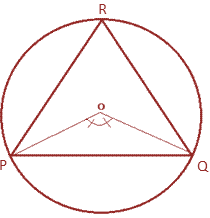Steps for constructions:

1. First draw a circle with centre O and radius = 3 cm.

2. Then draw radii OP and OB such that ∠POQ = 360/3 = 120o.

3. Now join PQ and cut off arcs PR and QR equal to PQ.

4. Join PR and QR.

Therefore, ΔABC is the required equilateral triangle.

15. Draw a circle of radius 2.5 cm and circumscribe a square about it.

Solution:-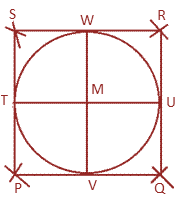Steps for constructions:

1. First draw a circle with centre M and radius = 2.5 cm.

2. Then draw two diameters TU and VW of the circle meeting at centre M.

3. Now taking MV as radius cut two arcs from V on both sides of V and repeat same with T, U, and W.

4. Then mark new points formed as P, Q, R and S.

5. So, join PQ, QR, RS and SP.

Therefore, PQRSC is the required square circumscribing the given circle.